### IMO Shortlist 1996 problem G9

Kvaliteta:
Avg: 0,0
Težina:
Avg: 10,0
In the plane, consider a point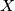$X$ and a polygon$\mathcal{F}$ (which is not necessarily convex). Let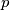$p$ denote the perimeter of$\mathcal{F}$, let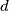$d$ be the sum of the distances from the point$X$ to the vertices of$\mathcal{F}$, and let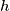$h$ be the sum of the distances from the point$X$ to the sidelines of$\mathcal{F}$. Prove that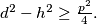$d^2 - h^2\geq\frac {p^2}{4}.$
Izvor: Međunarodna matematička olimpijada, shortlist 1996